## RS Aggarwal Class 7 Solutions Chapter 5 Exponents Ex 5C

These Solutions are part of RS Aggarwal Solutions Class 7. Here we have given RS Aggarwal Solutions Class 7 Chapter 5 Exponents Ex 5C.

Other Exercises

OBJECTIVE QUESTIONS
Mark (✓) tick against the correct answer in each of the following :
Question 1.
Solution:
(d)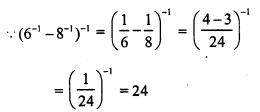Question 2.
Solution:
(c)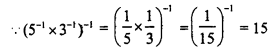Question 3.
Solution:
(c)Question 4.
Solution:
(b)Question 5.
Solution:
(c)Question 6.
Solution:
(b)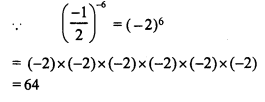Question 7.
Solution:
(b)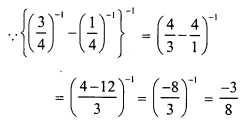Question 8.
Solution:
(a)Question 9.
Solution:
(c)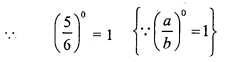Question 10.
Solution:
(b)Question 11.
Solution:
(b)Question 12.
Solution:
(b)Question 13.
Solution:
(d)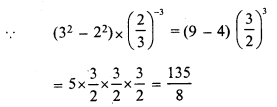Question 14.
Solution:
(a)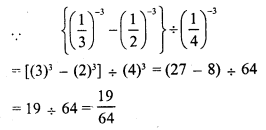Question 15.
Solution:
(c)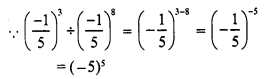Question 16.
Solution:
(a)Question 17.
Solution:
(c)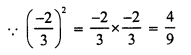Question 18.
Solution:
(b)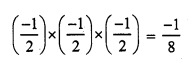Question 19.
Solution:
(c)Question 20.
Solution:
(c)
Required number = 10-1 ÷ (-8)-1Question 21.
Solution:
(c)
The number which is in standard form is 2.156 x 106

Hope given RS Aggarwal Solutions Class 7 Chapter 5 Exponents Ex 5C are helpful to complete your math homework.

If you have any doubts, please comment below. Learn Insta try to provide online math tutoring for you.